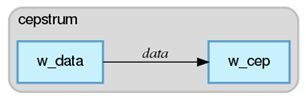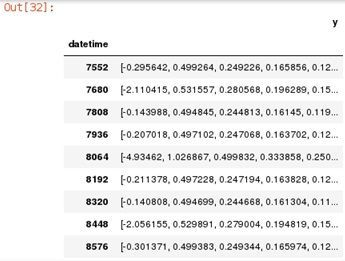## ESPPy: How to get started with the Python package for SAS Event Stream Processing

Started ‎11-14-2018 by
Modified ‎11-14-2018 by
Views 1,777

Let’s talk about ESPPy and how it fits into the SAS ESP framework. The development and testing of ESP projects has traditionally been done using the ESP Studio. There is now an additional way to develop and test ESP projects using Python. ESPPy is a Python package containing methods that allow the user to build and test ESP projects within a framework of Python code.

This should not be confused with the use of SAS Micro Analytic Service modules in ESP. In that case, the user can write Python code which is to be executed within an ESP project inside a Calculate Window. With this new ESPPy package, the entire ESP project can be developed in Python, including the use of MAS modules.

### Getting Started

We recommend that you use Anaconda as your Python environment because it is well suited for data science applications.

To install ESPPy, you can use either `pip` or `conda`. This will install ESPPy as well as the Python package dependencies.

``````pip install sas-esppy
``````

or, if you are using an Anaconda distribution:

``conda install -c sas-institute sas-esppy``

Aside from adding the location of this package to your Python search path, you’re done installing ESPPy. Your installation of Anaconda also comes with Jupyter Notebook. This is a very handy tool for developing in Python in general because you can run sections of your code individually, and you can visualize the results inside the notebook. Before trying to use ESPPy, you’ll need to install some other Python packages:

``````conda install pandas pillow ws4py requests
pip install graphviz``````

Whether you are using Jupyter Notebook, or just writing Python code in a text editor, you simply include this line at the beginning of your code:

``import esppy``

Before developing with ESPPy, it’s a good idea to run only the import statement to make sure that you have installed ESPPy correctly.

### Example: Cepstrum Analysis

Now that you have successfully installed ESPPy, let’s build a simple ESP project with Jupyter Notebook. This project will perform a cepstrum analysis on randomly generated input data. Cepstrum analysis is a very useful technique for signal processing, including human speech.

The first step is to make sure the desired packages can be imported and that the ESP server is available:

``````import esppy
import pandas as pd
import numpy as np
# connect to the esp server
esp = esppy.ESP('http://192.168.25.132:41001')``````

I encountered an issue when using ‘localhost’ instead of an ip address, so I recommend being explicit with the url. If that code block runs without error, we can create a project and a source window:

``````# Create a project
project = esp.create_project('cepstrum')

# Create a source window. The data has one id and one signal value.
# Add source window to the project
project.windows['w_data'] = src``````

Notice that we create the schema and specify the properties of the source window all in the same line. Once that is done, the next step is to add the window to the project. This is the Python equivalent of dragging and dropping a source window into the project canvas inside the ESP Studio.

The next steps are to create a Calculate window, which will use the cepstrum algorithm, and connect it to the source window we just created:

``````# Create a calculate window with the Cepstrum algorithm.
cep = esp.calculate.Cepstrum(schema=('datetime*:int64', 'y:array(dbl)'),
windowLength=128, windowType=7, overlap=0,
complexCepstrum=0,
input_map=dict(timeId='datetime',input='x'),
output_map=dict(
timeIdOut='datetime',
cepstrumListOut='y[1-128]'
))

# Add this window to the project
project.windows['w_cep'] = cep

# Add edges between windows to send data
# Visualize Project
project
# Generate XML for this project
print(project.to_xml(pretty=True))

# Load project into the serverThe process for creating the Calculate window is very much the same as for the Source window. Cepstrum is just one of the algorithms that is included with ESP and is a method that is used with the window object. As you can see, you can also visualize the project you are building as you go.

Now that we have created a working project, we can use the popular Python packages, numpy and pandas, to create sample data to stream into ESP:

``````# create fake data to publish to source window
def signal(time):
if 0 <= time < 5:
return np.cos(2*np.pi*10*time)
elif 5 <= time < 10:
return np.cos(2*np.pi*25*time)
elif 10 <= time < 15:
return np.cos(2*np.pi*50*time)
elif 15 <= time <= 20:
return np.cos(2*np.pi*100*time)
else:
raise ValueError("Input out of range!")

#sample the signal at 8kHz
t_id = np.arange(1,20*8000+1,1)
t = np.linspace(start=0, stop=20, num=20*8000)
x = np.vectorize(signal)(t)
#create pandas data frame
df = pd.DataFrame({'datetime':t_id, 'x':x})``````

Once we have loaded the sample data into a pandas dataframe, we can directly inject this dataframe into out Source window:

``````# Start publishing events from the dataframe
src.publish_events(df)``````

The above line of code is very simple, and that is a great thing. Data scientists often use dataframes to work with their data, and we have made the process of injecting dataframes into ESP as easy as can be.

We can subscribe to the various windows in our project like this:

``````# Subscribe with necessary windows
src.subscribe()
cep.subscribe()``````

And we can visualize the contents of a window as a table like this:

``cep.data``Conclusion

ESPPy is a great Python package for data scientists to use that combines many familiar tools with the capabilities of SAS ESP.

An API Reference Guide is available here:

https://sassoftware.github.io/python-esppy/api.html

Version history
Last update:
‎11-14-2018 02:32 PM
Updated by:Contributors
Article Labels
Article Tags